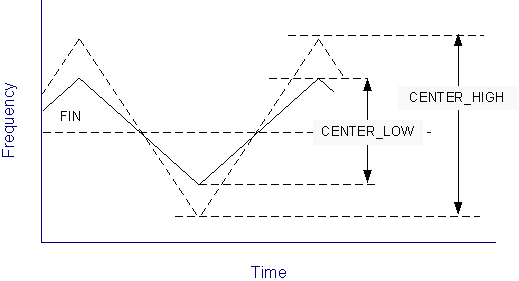# AR# 51561

|

## 描述fig1.pngfig2.png

When using spread spectrum in SS_MODE(CENTER_HIGH) or SS_MODE( CENTER_LOW), the center spread will increase the effective frequency ranges.
No versions of ISE Design Suite support automatic timing analysis for CENTER_HIGH or CENTER_LOW. Current versions Vivado Design Suite also do not support automatic timing analysis for CENTER_HIGH or CENTER_LOW.

## 解决方案

The user must manually constrain the input clock to account for the range of frequencies within the Spread Spectrum.

The table below gives the input frequency adjustment formula (FIN_SS) that is required based on the Spread Spectrum modes selected (SS_MODE), the Input Frequency (FIn) and the Multiply value selected (M).

 Input Frequency [MHz] M Input Frequency Adjustment (FIN_SS) SS_MODE(CENTER_HIGH) 25 < FIN < 35 M=28 FIN_SS = FIN * 56 / 55 35 < FIN < 50 M=21 FIN_SS = FIN * 42 / 41 M=22 FIN_SS = FIN * 44 / 43 50 < FIN < 75 M=28 FIN_SS = FIN * 56 / 55 75 < FIN < 150 M=21 FIN_SS = FIN * 42 / 41 M=22 FIN_SS = FIN * 44 / 43 SS_MODE (CENTER_LOW) 25 < FIN < 35 M=56 FIN_SS = FIN * 112 / 111 35 < FIN < 50 M=42 FIN_SS = FIN * 84 / 83 M=44 FIN_SS = FIN * 88 / 87 50 < FIN < 75 M=56 FIN_SS = FIN * 112 / 111 75 < FIN < 150 M=42 FIN_SS = FIN * 84 / 83 M=44 FIN_SS = FIN * 88 / 87 SS_MODE (DOWN_HIGH) 25 < FIN < 35 M=28 FIN_SS = FIN 35 < FIN < 50 M=21, 22 FIN_SS = FIN 50 < FIN < 75 M=28 FIN_SS = FIN 75 < FIN < 100 M=21, 22 FIN_SS = FIN 100 < FIN < 150 M=21, 22 FIN_SS = FIN SS_MODE (DOWN_LOW) 25 < FIN < 35 M=56 FIN_SS = FIN 35 < FIN < 50 M=42, 44 FIN_SS = FIN 50 < FIN < 75 M=56 FIN_SS = FIN 75 < FIN < 100 M=42, 44 FIN_SS = FIN 100 < FIN < 150 M=42, 44 FIN_SS = FIN

With a 25 MHz input clockthenew timing constraint would be as follows:

SS_MODE(CENTER_HIGH) = 25 * 56/55 = 25.45 MHz

SS_MODE (CENTER_LOW) = 25 * 112/111 = 25.23 MHz

SS_MODE (DOWN_HIGH) and SS_MODE (DOWN_LOW) as the Spread will reduce the frequency, then no change is needed to the input Clock.

With an 80 MHz clock with M = 22 for (CENTER_HIGH) and M = 44 for (CENTER_LOW):

SS_MODE(CENTER_HIGH) =80 *44/43 = 81.86 MHz

SS_MODE (CENTER_LOW) =80*88/87 = 80.92 MHz

Again, as for SS_MODE (DOWN_HIGH) and SS_MODE (DOWN_LOW), as the Spread will reduce the frequency, then no change is needed to the input Clock.

The above will be required for all versions of ISE Design Suite. Vivado Design Suite 2013.1onwards has support for adjusting the timing constraints automaticallybased on the Spread Spectrum clocking usage.